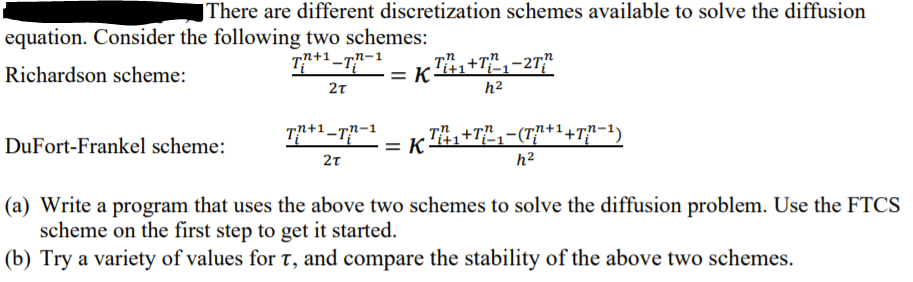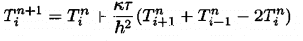# Matlab coding issue with solving PDEs

Homework Statement:
I was tasked with putting a few methods for solving PDEs into matlab. I started with the given code that solved using FTCS method. Below is was i have modified it to. I understand i need to have it run FTCS for the first step. I am not sure if the way i have written the equations into the code makes sense but i have gotten the Richardson method to produce results. There is an error with the Dufort method though
"Array indices must be positive integers or
logical values.

Error in problem3 (line 45)
tt(2:(N-1)) = tt(2:(N-1)) +
2*coeff(tt(3:N) + tt(1:(N-2)) -
tt(2:(N-1)))/(1+ 2*coeff);
"
Relevant Equations:
FTCS equation is the same form is copied below from my textFTCS schemeMy Matlab code:
%Problem 3
%Solve diffusion problem using Richardson scheme or DuFort-Frankel scheme
clear all;

scheme = menu('Choose method of solving diffusion equation:', 'Richardson', 'DuFort-Frankel');
tau = input('Enter time step: ');
N = input('Enter the number of grid points: ');
L = 1.; % The system extends from x=-L/2 to x=L/2
h = L/(N-1); % Grid size
k = 1.; % Diffusion coefficient
coeff = k*tau/h^2;

if( coeff < 0.5 )
disp('Solution is expected to be stable');
else
disp('WARNING: Solution is expected to be unstable');
end
%* Set initial and boundary conditions.
tt = zeros(N,1); % Initialize temperature to zero at all points
tt(round(N/2)) = 1/h; % Initial cond. is delta function in center
% The boundary conditions are tt(1) = tt(N) = 0
%* Set up loop and plot variables.
xplot = (0:N-1)*h - L/2; % Record the x scale for plots
iplot = 1; % Counter used to count plots
nstep = 300; % Maximum number of iterations
nplots = 50; % Number of snapshots (plots) to take
plot_step = nstep/nplots; % Number of time steps between plots

%Run First steps using FTCS
tt(2:(N-1)) = tt(2:(N-1)) + coeff*(tt(3:N) + tt(1:(N-2)) - 2*tt(2:(N-1)))
%Run selected scheme
if (scheme==1) %richardson
for istep=2:nstep
tt(2:(N-1)) = tt(2:(N-1)) + 2*coeff*(tt(3:N) + tt(1:(N-2)) - 2*tt(2:(N-1)));

if( rem(istep,plot_step) < 1 ) % Every plot_step steps
ttplot(:,iplot) = tt(:); % record tt(i) for plotting
tplot(iplot) = istep*tau; % Record time for plots
iplot = iplot+1;
end
end
else (scheme==2)%dufort
for istep=2:nstep
tt(2:(N-1)) = tt(2:(N-1)) + 2*coeff(tt(3:N) + tt(1:(N-2)) - tt(2:(N-1)))/(1+ 2*coeff);

if( rem(istep,plot_step) < 1 ) % Every plot_step steps
ttplot(:,iplot) = tt(:); % record tt(i) for plotting
tplot(iplot) = istep*tau; % Record time for plots
iplot = iplot+1;
end
end
end

%* Plot temperature versus x and t as wire-mesh and contour plots.
figure(1); clf;
mesh(tplot,xplot,ttplot); % Wire-mesh surface plot
xlabel('Time'); ylabel('x'); zlabel('T(x,t)');
title('Diffusion of a delta spike');
pause(1);
figure(2); clf;
contourLevels = 0:0.5:10; contourLabels = 0:5;
cs = contour(tplot,xplot,ttplot,contourLevels); % Contour plot
clabel(cs,contourLabels); % Add labels to selected contour levels
xlabel('Time'); ylabel('x');
title('Temperature contour plot');

## Answers and Replies

Tom.G
Science Advisor
"Array indices must be positive integers...

Error in problem3 (line 45)
tt(2:(N-1)) = tt(2:(N-1)) +
2*coeff(tt(3:N) + tt(1:(N-2)) -
tt(2:(N-1)))/(1+ 2*coeff);
"

for istep=2:nstep
tt(2:(N-1)) = tt(2:(N-1)) + 2*coeff*(tt(3:N) + tt(1:(N-2)) - 2*tt(2:(N-1)));